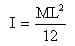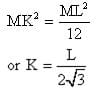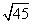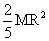NEET  >  Test: Moment of Inertia

# Test: Moment of Inertia

Test Description

## 10 Questions MCQ Test Physics Class 11 | Test: Moment of Inertia

Test: Moment of Inertia for NEET 2022 is part of Physics Class 11 preparation. The Test: Moment of Inertia questions and answers have been prepared according to the NEET exam syllabus.The Test: Moment of Inertia MCQs are made for NEET 2022 Exam. Find important definitions, questions, notes, meanings, examples, exercises, MCQs and online tests for Test: Moment of Inertia below.
Solutions of Test: Moment of Inertia questions in English are available as part of our Physics Class 11 for NEET & Test: Moment of Inertia solutions in Hindi for Physics Class 11 course. Download more important topics, notes, lectures and mock test series for NEET Exam by signing up for free. Attempt Test: Moment of Inertia | 10 questions in 10 minutes | Mock test for NEET preparation | Free important questions MCQ to study Physics Class 11 for NEET Exam | Download free PDF with solutions
 1 Crore+ students have signed up on EduRev. Have you?
Test: Moment of Inertia - Question 1

### The radius of gyration of a ring of radius R about an axis through its centre and perpendicular to its plane is

Detailed Solution for Test: Moment of Inertia - Question 1

For a ring moment of inertia ,I=M*R*R   M=Mass and R=Radius

In terms of radius of gyration

I=M*K*K

M*R*R=M*K*K

Therefore ,K=R

Test: Moment of Inertia - Question 2

### Two rings have their moment of inertia in the ratio 2:1 and their diameters are in the ratio 2:1. The ratio of their masses will be:

Detailed Solution for Test: Moment of Inertia - Question 2

We know that MI of a ring is mr2
Where m is mass of the ring and r is its radius
When we have ratio of I = 2:1
And ratio of r = 2:1
We get ratio of r2 = 4:1
Thus to make this ratio 2:1 , that ratio of masses must be 1:2

Test: Moment of Inertia - Question 3

### The radius of gyration of uniform rod of length L and mass M about an axis passing through its centre and perpendicular to its length is

Detailed Solution for Test: Moment of Inertia - Question 3

If K is radius of gyration, then moment of inertia for the rod is given as -

I = MK2                        (1)

But moment of inertia of uniform rod of length L and Mass M about an axis passing through its center the perpendicular to its length  is given as -comparing equation (1) and (2), we getTest: Moment of Inertia - Question 4

Moment of inertia of two spheres of equal radii are equal. One of the spheres is solid and has the mass 5 kg and the other is a hollow sphere. What is the mass of hollow sphere?

Detailed Solution for Test: Moment of Inertia - Question 4

We know that for a solid sphere of mass S and radius r, moment of inertia I = ⅖ Sr2
Similarly for a hollow sphere of mass H and radius r, moment of inertia I = ⅔ Hr
Thus if there radii and I are equal the ratio of there mass can be find by equating both as
I =  ⅖ Sr2  = ⅔ Hr2
Hence we het H/S = ⅗
So if S is 5kg we get H = 3kg

Test: Moment of Inertia - Question 5

A solid cylinder of 200g and radius 10 cm has a moment of inertia (about its natural axis)

Detailed Solution for Test: Moment of Inertia - Question 5

Moment of inertia of solid cylinder
MR2/2=0.2*10*10-2*10*10-2  Kg m2
=2*10-3/2
=10-3 Kg m2

Test: Moment of Inertia - Question 6

There are two circular iron discs A and B having masses in the ratio 1:2 and diameter in the ratio 2:1. The ratio of their moment of inertia is

Detailed Solution for Test: Moment of Inertia - Question 6

Given,
Mass of A=1,
Mass of B=2.
diameter if A=2,
diameter if B=1.
radius (r) of A=d/2=2/2=1.
radius (r) of B=d/2=1/2.
we know ,
moment of inertia of disc=MR2/2.
moment of inertia (I)of A/moment of inertia (I)of B=MR2/2/MR2/2.
(I) of A/(I) of B=1×12/2/2×(1/2)2/2.
=1×1/2/2×(1/4)/2.
=1/2/(1/2)/2.
=1/2/1/4.
=4/2.
=2/1.

Test: Moment of Inertia - Question 7

The moment of inertia of two spheres of equal masses is equal. If one of the spheres is solid of radiusm and the other is a hollow sphere. What is the radius of the hollow sphere?

Detailed Solution for Test: Moment of Inertia - Question 7

Moment of inertia of solid sphere Is= 2/5MR2
moment of inertia of hollow sphere Ih =2/3MR2
given mass of solid sphere =√45 kg.
Is=Ih
2MR2/5=2MR2/3
given their masses are equal 2 (√45)2/5= 2 R2/3
45/5=R2/3
9=R2/3
9×3=R2
27=R2
√27=R
√3×9=R
3√3 m=R.

Test: Moment of Inertia - Question 8

The moment of inertia of a body is independent of

Detailed Solution for Test: Moment of Inertia - Question 8

The moment of inertia of a body is independent of. The moment of inertia of a body is independent of its angular velocity.It depends upon the position and orientation of the axis of rotation,shape and size of the body and distribution of mass of the body about the axis of rotation.

Test: Moment of Inertia - Question 9

The moment of inertia of a solid sphere about its diameter is.Find moment of inertia about its tangent.

Detailed Solution for Test: Moment of Inertia - Question 9

The moment of inertia (M.I.) of a sphere about its diameter=2MR2/5
According to the theorem of parallel axes, the moment of inertia of a body about any axis is equal to the sum of the moment of inertia of the body about a parallel axis passing through its centre of mass and the product of its mass and the square of the distance between the two parallel axes.
The M.I. about a tangent of the sphere =2MR2/5+MR2=7MR2/5

Test: Moment of Inertia - Question 10

A ring has greater moment of inertia than a circular disc of same mass and radius, about an axis passing through its centre of mass perpendicular to its plane, because

Detailed Solution for Test: Moment of Inertia - Question 10

A ring has a larger moment of inertia because its entire mass is concentrated at the rim at maximum distance from the axis.

## Physics Class 11

126 videos|449 docs|210 tests
 Use Code STAYHOME200 and get INR 200 additional OFF Use Coupon Code
Information about Test: Moment of Inertia Page
In this test you can find the Exam questions for Test: Moment of Inertia solved & explained in the simplest way possible. Besides giving Questions and answers for Test: Moment of Inertia, EduRev gives you an ample number of Online tests for practice

## Physics Class 11

126 videos|449 docs|210 tests

### How to Prepare for NEET

Read our guide to prepare for NEET which is created by Toppers & the best Teachers